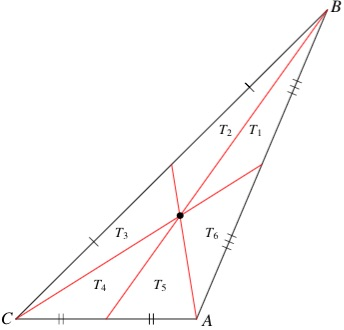# Similar Triangles in a Triangle

This diagram shows a triangle divided into six triangles T_i by the three medians:Denote similarity by ~. An equilateral triangle has the property that

T1 ~ T1 + T2 + T3
T2 ~ T2 + T3 + T4
T3 ~ T1 + T5 + T6
T4 ~ T1 + T2 + T6
T5 ~ T3 + T4 + T5
T6 ~ T4 + T5 + T6

Find the smallest nonequilateral triangle with integer sides that satisfies these six similarity conditions. Smallest means that the perimeter is smallest.

Extra Credit: Investigate the general situation. Are there other ways of arranging these similarities (naively there are 720 possible ways to do it, based on the permutations of the right sides) that lead to non-equilateral solutions.

Source: Lee Sallows and Stan Wagon.

23 March 2015